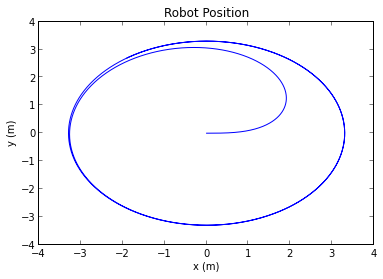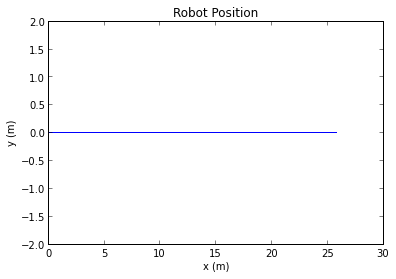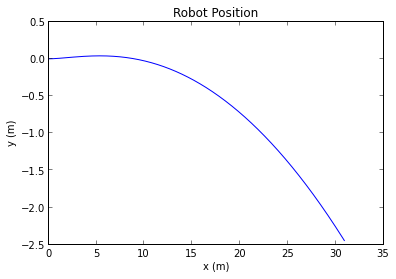# Differential Drive Dynamics - Part 1

Posted on March 16, 2014

# Differential Drive Robotics Platform¶

I've been using SymPy in my research and coursework for a while now. For those that don't know, SymPy is a computer algebra system, capable of performing symbolic calculations that would be too complicated to do by hand. Which makes it perfect for solving the equations needed to generate the equations of motion (EOM) of multibody systems! In this post, I'll demonstrate a simple workflow for generating the EOM for a differential drive robot.

## Problem Setup¶

A common robot platform is that of the differential drive. The robot is propelled using two wheels, each with their own motor. Usually a caster is used as a third wheel to provide stability. By varying the speeds of the two motors, the robot can change direction.

Differential drive platforms are highly maneuverable, as they have a very small turn radius. They are also extremely simple to design. In this example, the dynamics of a differential drive platform will be explored. The resulting model is highly accurate, but makes a few assumptions:

Assumptions

• Wheels roll without slipping
• Body is symmetric

The setup is as follows:

In :
from IPython.display import SVG
SVG(filename='Robot.svg')

Out:

## Equations of Motion¶

We’ll start by generating the equations of motion for the robot with sympy.physics.mechanics. The mechanics module provides a clean, intuitive workflow for deriving the equations of motion for multibody dynamic systems using either KanesMethod or LagrangesMethod objects. In this example we'll make use of the LagrangesMethod object, which generates the equations of motion using Lagrangian Mechanics with constraints and generalized forces.

First we import the necessary functionality from SymPy.

In :
from sympy.physics.mechanics import *
from sympy import sin, cos, symbols, Matrix, solve


Next we need to define an inertial reference frame, as well as a coordinate origin. All coordinates and reference frames defined later will be in reference to the world coordinate frame.

In :
#Inertial Reference Frame
N = ReferenceFrame('N')

#Define a world coordinate origin
O = Point('O')
O.set_vel(N, 0)


Lagrange's Method requires a state vector $\vec{q}$ that contains all the generalized coordinates required to define the system in space. In the case of the robot:

$$\vec{q} = [\matrix{x && y && \theta && \phi_1 && \phi_2}]^T$$

As these are all time varying variables, they need to be created as dynamicsymbols:

In :
theta = dynamicsymbols('theta')                      #Rotation about N.z
x, y = dynamicsymbols('x, y')                        #Coordinates of robot in World Frame
phi1, phi2 = dynamicsymbols('phi_1, phi_2')          #Angular displacement of wheel

#Create q and dq vectors
q = Matrix([x, y, theta, phi1, phi2])
dq = q.diff()


We'll also define some constant parameters that define the specific robot:

In :
#Constants for the wheels
r = symbols('r')                                     #Radius of wheel
m_w = symbols('m_w')                                 #Mass of the wheel
t_w = symbols('t_w')                                 #Thickness of the wheel

#Constants for the Robot Body
w = symbols('w')                                     #2*w is the width of the wheel base
d = symbols('d')                                     #Distance between axel and center of mass
m_b = symbols('m_b')                                 #Mass of the body
Ixx, Iyy, Izz = symbols('Ixx, Iyy, Izz')             #Moments of inertia of body


Next we need to define a reference frame relative to the robot, and a point located at the center of the wheel axis. This will define the robot's location in the world coordinate frame. The velocity of this point is just the derivative of its x and y coordinates.

In :
#Robot Reference Frame
R = N.orientnew('R', 'Axis', [theta, N.z])

#Center of wheel base
Cw = O.locatenew('Cw', x*N.x + y*N.y)

#Set the velocity of point Cw
Cw.set_vel(N, x.diff()*N.x + y.diff()*N.y)


Coordinates of the 2 wheel hubs then need to be specified. Luckily sympy mechanics makes this easy to do with the locatenew method. The hubs 1 and 2 are located at $-w\vec{R_y}$ and $w\vec{R_y}$ away from the center of the wheel base $C_w$ respectively.

The velocity of the hubs is then found using the v2pt_theory method, which calculates the velocity of a point based off the velocity of another point in the same frame, and the angular velocity of that frame (reference).

In :
#Points at wheel hubs
H1 = Cw.locatenew('H1', -w*R.y)
H2 = Cw.locatenew('H2', w*R.y)

#Set the velocity of points H1 and H2
H1.v2pt_theory(Cw, N, R)
H2.v2pt_theory(Cw, N, R);


Rotating reference frames fixed to each wheel are then created. These rotate about the $\vec{R_y}$ axis with the angular position of the wheels, $\phi_1$ and $\phi_2$.

In :
#Create reference frames for wheels 1 and 2
W1 = R.orientnew('W1', 'Axis', [phi1, R.y])
W2 = R.orientnew('W2', 'Axis', [phi2, R.y])


sympy.physics.mechanics provides classes for rigid bodies. These make calculating the lagrangian of a multibody system easier, as each body can be handled separately. To do this, each body needs the following things specified:

• A name
• A center of mass
• A reference frame
• A mass
• A tuple of (Inertia, Point of rotation)

Modeling the wheels as solid cylinders, the inertia can be calculated about the hubs, and RigidBody objects can be created for each wheel.

In :
#Calculate inertia of the wheel
Iw = inertia(R, m_w*(3*r**2 + t_w**2)/12, m_w*r**2/2, m_w*(3*r**2 + t_w**2)/12)

#Create rigid bodies for wheels
Wheel1 = RigidBody('Wheel1', H1, W1, m_w, (Iw, H1))
Wheel2 = RigidBody('Wheel2', H2, W2, m_w, (Iw, H2))


The same can be done for the body of the robot. In this case we model the body as a symmetric object with inertias $I_{xx}$, $I_{yy}$, and $I_{zz}$. The center of mass of the body is located a distance $d$ in front of the wheel axel.

In :
#Calculate inertia of body
Ib = inertia(R, Ixx, Iyy, Izz)

#Center of mass of body
Cm = Cw.locatenew('Cm', d*R.x)
Cm.v2pt_theory(Cw, N, R)

#Create a rigid body object for body
Body = RigidBody('Body', Cm, R, m_b, (Ib, Cm))


## Nonholonomic Constraints¶

At this point, the lagrangian of the system can be calculated. However, the system still isn't fully defined; the constraints still need to be specificed.

At this instance in time, the velocity of the ground contact points must have a velocity of zero. To specify this in the lagrange equations, a constraint matrix needs to be created.

First, two points are created where the wheels contact the ground, and their velocities calculated.

In :
#Create two points, where the wheels contact the ground
C1 = H1.locatenew('C1', -r*R.z)
C2 = H2.locatenew('C2', -r*R.z)
#Calculate velocity of points
C1.v2pt_theory(H1, N, W1)
C2.v2pt_theory(H2, N, W2);


Using these velocities, a system of equations can be created. As the velocities must be zero, this expression = 0.

In :
#Express the velocity of points in the inertial frame
con1 = C1.vel(N).express(N).args
con2 = C2.vel(N).express(N).args
#Create a matrix of constraints
constraints = con1.col_join(con2)
mprint(constraints)

Matrix([
[(-r*phi_1' + w*theta')*cos(theta) + x'],
[(-r*phi_1' + w*theta')*sin(theta) + y'],
[                                     0],
[(-r*phi_2' - w*theta')*cos(theta) + x'],
[(-r*phi_2' - w*theta')*sin(theta) + y'],
[                                     0]])


There are 6 equations with 3 unknowns ($\dot{x}$, $\dot{y}$, and $\dot{theta}$), there are duplicate equations. Using solve, and reconfiguring the expression, this can be reduced to the required set:

In :
#Solve for dx, dy, and dtheta in terms of dphi1 and dphi2
sol = solve(constraints, dq[:3])

#Split the resulting dict into a rhs and lhs, that are equivalent
sol_rhs = Matrix(sol.values())
sol_lhs = Matrix(sol.keys())

#Since sol_rhs = sol_lhs --> sol_rhs - sol_lhs = 0
#This forms the basis of our constraint matrix.
#Combining, and solving for a linear representation:
c = (sol_rhs - sol_lhs).jacobian(dq[:5])
mprint(c)

Matrix([
[-1,  0,  0, r*cos(theta)/2, r*cos(theta)/2],
[ 0,  0, -1,        r/(2*w),       -r/(2*w)],
[ 0, -1,  0, r*sin(theta)/2, r*sin(theta)/2]])


This is our constraint matrix. The LagrangesMethod class requires that the constraints be specified as

coneqs = $C\dot{\vec{q}} = 0$

Thus:

In :
#Constraint Equations
coneqs = (c*dq)
mprint(coneqs)

Matrix([
[r*cos(theta)*phi_1'/2 + r*cos(theta)*phi_2'/2 - x'],
[          r*phi_1'/(2*w) - r*phi_2'/(2*w) - theta'],
[r*sin(theta)*phi_1'/2 + r*sin(theta)*phi_2'/2 - y']])


## Generalized Forces¶

The inputs of system are the torques at the wheels. Using the right-hand-rule, it can be seen that a positive torque will exert force on the system in the $R_x$ direction. With a wheel radius of $r$, the force on the wheel hubs is:

$\vec{F_{hub}} = \tau*\vec{R_x}$

In :
#Define forces on system:
T1, T2 = symbols('tau_1, tau_2')              #Torques from the wheels
fl = [(H1, r*T1*R.x),
(H2, r*T2*R.x)]


## Solving the System Dynamics¶

We are now ready to solve the for the equations of motion. First, calculate the lagrangian of the system $L = T - V$:

In :
Lag = Lagrangian(N, Wheel1, Wheel2, Body)


Next, a LagrangesMethod object is created. This takes the following parameters:

• The system lagrangian
• The generalized coordinate vector $\vec{q}$
• The constraint equations
• A list force tuples: (Point, Force at point)
• An inertial reference frame
In :
lm = LagrangesMethod(Lag, q, coneqs=coneqs, forcelist=fl, frame=N)


The equations of motion can then be found:

In :
le = lm.form_lagranges_equations()
mprint(le)

Matrix([
[                                                                                                                                                                                                                                                                                                                                                                                                                                                                                                                                                                                                                                                                                                                                                     m_b*(-2*d*sin(theta)*theta'' - 2*d*cos(theta)*theta'**2 + 2*x'')/2 + m_w*(-2*w*sin(theta)*theta'**2 + 2*w*cos(theta)*theta'' + 2*x'')/2 + m_w*(2*w*sin(theta)*theta'**2 - 2*w*cos(theta)*theta'' + 2*x'')/2 - r*tau_1*cos(theta) - r*tau_2*cos(theta) - lam1],
[                                                                                                                                                                                                                                                                                                                                                                                                                                                                                                                                                                                                                                                                                                                                                     m_b*(-2*d*sin(theta)*theta'**2 + 2*d*cos(theta)*theta'' + 2*y'')/2 + m_w*(-2*w*sin(theta)*theta'' - 2*w*cos(theta)*theta'**2 + 2*y'')/2 + m_w*(2*w*sin(theta)*theta'' + 2*w*cos(theta)*theta'**2 + 2*y'')/2 - r*tau_1*sin(theta) - r*tau_2*sin(theta) - lam3],
[Izz*theta'' - m_b*(d*(-sin(theta)*y' - cos(theta)*x')*theta' - d*sin(theta)*theta'*y' - d*cos(theta)*theta'*x')/2 + m_b*(2*d**2*theta'' + d*(-sin(theta)*theta'*y' - sin(theta)*x'' - cos(theta)*theta'*x' + cos(theta)*y'') - d*sin(theta)*theta'*y' - d*sin(theta)*x'' - d*cos(theta)*theta'*x' + d*cos(theta)*y'')/2 + m_w*(3*r**2 + t_w**2)*theta''/6 - m_w*(-w*(-sin(theta)*x' + cos(theta)*y')*theta' + w*sin(theta)*theta'*x' - w*cos(theta)*theta'*y')/2 - m_w*(w*(-sin(theta)*x' + cos(theta)*y')*theta' - w*sin(theta)*theta'*x' + w*cos(theta)*theta'*y')/2 + m_w*(2*w**2*theta'' - w*(-sin(theta)*theta'*x' + sin(theta)*y'' + cos(theta)*theta'*y' + cos(theta)*x'') + w*sin(theta)*theta'*x' - w*sin(theta)*y'' - w*cos(theta)*theta'*y' - w*cos(theta)*x'')/2 + m_w*(2*w**2*theta'' + w*(-sin(theta)*theta'*x' + sin(theta)*y'' + cos(theta)*theta'*y' + cos(theta)*x'') - w*sin(theta)*theta'*x' + w*sin(theta)*y'' + w*cos(theta)*theta'*y' + w*cos(theta)*x'')/2 - r*tau_1*w + r*tau_2*w - lam2],
[                                                                                                                                                                                                                                                                                                                                                                                                                                                                                                                                                                                                                                                                                                                                                                                                                                                                                                                                    m_w*r**2*phi_1''/2 + r*lam1*cos(theta)/2 + r*lam3*sin(theta)/2 + r*lam2/(2*w)],
[                                                                                                                                                                                                                                                                                                                                                                                                                                                                                                                                                                                                                                                                                                                                                                                                                                                                                                                                    m_w*r**2*phi_2''/2 + r*lam1*cos(theta)/2 + r*lam3*sin(theta)/2 - r*lam2/(2*w)]])


Sympy makes this super easy: no need to do this by hand, and no more mistakes in algebra. However, these are rather complicated equations. Luckily, they can be converted into a function allowing numerical simulation.

## Simulation¶

To gain insight into the system dynamics, numerical simulation will be used. First, we need to import a few more functions:

In :
from sympy import lambdify, Dummy
from scipy import array, hstack, linspace, ones
from scipy import random, interp, vstack
from scipy.integrate import odeint
import matplotlib.pyplot as plt
%matplotlib inline


The vector $\ddot{\vec{q}}$ can be calculated in a closed form expression using the rhs method. This method takes quite a long time to solve entirely symbolically (it took over an hour on my machine), so a simplifying assumption will be made: $d = 0$. This is actually pretty reasonable, as the motors and battery will be the heaviest part, and should be situated around the axle.

In :
#Substitute in d = 0
lm.eom = lm.eom.subs({d: 0})

#Solve for the rhs:
rhs = lm.rhs()


We're almost done, all we need to do is model the dynamics of the motor. In the future there may be dynamics classes for electronics, allowing a piecewise construction like we did for the mechanics (using RigidBody). Defining the motor dynamics using the current code-base isn't terribly tricky though.

In general, we can model the current dynamics of the motor as follows:

$$\dot{i} = -K/L \dot{\phi} - R/L i + V/L$$$$\tau = K i$$

where $i$ and $V$ are the current and voltage through the motor, $K$ is the motor constant, $R$ is the coil resistance, and $L$ is the coil inductance. Creating this model in sympy is fairly straightforward:

In :
#Create dynamic symbols for current and voltage
i_1, i_2 = dynamicsymbols('i_1, i_2')        #Currents through motor 1 and 2
V_1, V_2 = symbols('V_1, V_2')               #Voltages across the motor terminals

#Define some motor constants.
#Assuming motor 1 and 2 are the same:
R = symbols('R')                             #Coil resistance
L = symbols('L')                             #Coil inductance
K1, K2 = symbols('K1, K2')                   #Motor constant

#Define the motor dynamics
di = Matrix([[-K1/L*phi1.diff() - R/L*i_1 + V_1/L],
[-K2/L*phi2.diff() - R/L*i_2 + V_2/L]])


Now we just need to combine the motor model with the model for the robot:

In :
#Define consts:
params = [Izz,  t_w,  m_w,    r,  m_b,   w,    R,      L,   K1,  K2]
values = [  5, 0.15,  2.0, 0.15, 50.0, 0.6, 0.05, 0.0001,  1.0, 1.0]       #Values of constants

#Create a list of dynamic symbols for simulation
dynamics = q.T.tolist() + dq.T.tolist() + lm.lam_vec.T.tolist() + [i_1, i_2]

#Set the inputs to be the motor voltages
inputs = [V_1, V_2]

#Add the motor model to the rhs equations
aug_rhs = rhs.col_join(di)

#Substitute in K*i for T in the rhs equations
aug_rhs = aug_rhs.subs({T1: K1*i_1, T2: K2*i_2})


This can now be converted into a function to solve the rhs based on current state using lambdify:

In :
#Create a list of dynamic symbols for simulation
dummys = [Dummy() for i in dynamics]
dummydict = dict(zip(dynamics, dummys))
#Sub in the dummy symbols
rhs_dummy = aug_rhs.subs(dummydict)
#Lambdify function
rhs_func = lambdify(dummys + inputs + params, rhs_dummy)

In :
#Create a function in the required format for odeint
def right_hand_side(x, t, ts, us, values):
"""Calculate the rhs of the integral, at
time t, state x.
ts, us are used to get the current input
values are constants in the integral"""

#Interp is needed to get u for timestep
u1 = interp(t, ts, us[:,0])
u2 = interp(t, ts, us[:,1])

arguments = hstack((x, u1, u2, values))

#Need call to array and reshape, as odeint
#requires state vector to be 1d
dx = array(rhs_func(*arguments))
return dx.reshape(dx.size)


Now we have everything we need to simulate the motion. First, let's see what happens if we input a constant, unbalanced voltage signal. Motor 1 gets 24 volts, Motor 2 gets 20 volts. Intuitively, this should cause the robot to drive in a circle.

In :
ts = linspace(0, 30, 3000)
us = vstack((24*ones(len(ts)), 20*ones(len(ts)))).T
#Run the simulation
x0 = [0,] * 15                         #Start out at origin, unmoving
xs = odeint(right_hand_side, x0, ts, args=(ts, us, values))

plt.plot(xs[:,5], xs[:,6])
plt.title('Robot Position')
plt.xlabel('x (m)')
plt.ylabel('y (m)');As seen by the simulation, the results line up with our intuition. The reason it doesn't immediately start out in a circle is due to the time it takes for it to accelerate from rest.

Next, lets see what happens when the input voltages are matched:

In :
ts = linspace(0, 10, 1000)
us = 20*ones((len(ts),2))
#Run the simulation
x0 = [0,] * 15                         #Start out at origin, unmoving
xs = odeint(right_hand_side, x0, ts, args=(ts, us, values))
plt.plot(xs[:,0], xs[:,1])
plt.title('Robot Position')
plt.xlabel('x (m)')
plt.ylabel('y (m)');
plt.ylim(-2, 2);As expected, the robot drives in a straight line.

## Simulation - Need for control¶

Currently our robot is driven in "open-loop" control. This means that an input signal is sent, but no output is measured. This is fine if our model is perfect, and there are no disturbances. But the real world isn't like that. For example, what if our motors aren't perfectly balanced, and had slightly different K values?

To see, we'll change the K value of Motor 2 to 0.99. Just a small difference.

In :
#Change the value of K_2
values = [5, 0.15, 2.0, 0.15, 50.0, 0.6, 0.05, 0.0001, 1.0, 0.99]

#Re-run the straight line simulation
ts = linspace(0, 10, 1000)
us = 24*ones((len(ts),2))

#Run the simulation
x0 = [0,] * 15                         #Start out at origin, unmoving
xs = odeint(right_hand_side, x0, ts, args=(ts, us, values))
plt.plot(xs[:,0], xs[:,1])
plt.title('Robot Position')
plt.xlabel('x (m)')
plt.ylabel('y (m)');After 10 seconds, the robot is already 2.5 m off it's intended course! Luckily, a simple feedback controller should be able to solve this.

As our model is highly nonlinear, the first thing that should be done is to linearize it about our setpoint. However, currently the LagrangesMethod class doesn't have a linearization method. And because there are constraints, simply computing the Jacobian of the RHS isn't a valid solution. So an LQR controller, or other traditional state space design methods are out.

To develop a controller for this robot, a simple linear model would need to be developed. This will be covered in part 2, but for now, let's just save the model to file so it can be opened later without rederiving:

In :
#srepr returns the python code needed to recreate a
#sympy expression
from sympy.printing import srepr

def save_sol(expr, file_path):
"""Save a sympy expression to file

To reload the expression:

import file_path

"""

fil = open(file_path, "w")
fil.write("from sympy import *\n")
fil.write(srepr(expr))
fil.write("\n    return expression")
fil.close()

In :
#Save the rhs for later evaluation
save_sol(rhs, "robot_rhs.py")


This can later be reloaded by calling:

import robot_rhs

Overall, the sympy.physics.mechanics workflow for modeling multibody dynamics is easy to use. As shown above, the tools for generating a model based off of its components (wheels, body, etc...), solving for the system dynamics, and then simulating the resulting expression are provided in an intuitive manner. While larger/more complicated models may take some time (I've gotten the n-pendulum example to solve for up to 25 links), the resulting expression in symbolic form allows for simulation and analysis in a way that traditional numerical methods cannot provide. With some improvements to the simplification routines, and the addition of a linearization routine the hope is that the complicated expressions shown here can be simplified to "human readable form". This would allow for fast run-time due to simplified expressions, as well as easier analysis.
Note: The code used here was developed as part of the pydy project. Other awesome portions of the pydy workflow have not been demonstrated here. Some work is currently being done on code generation to generate code in C, FORTRAN, or other language from a sympy expression. This would allow for faster simulations, and easy integration into embedded controllers or estimators. There is also some work being done on visualization, resulting in an animation of the system dynamics running in a browser.